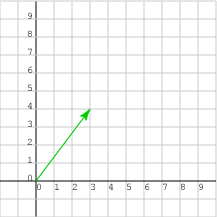created 08/26/97; revised 08/12/00, 07/22/03, 07/22/09, 01/11/15

# CHAPTER 6 — Scaling and Unit VectorsThis chapter discusses how to multiply a vector by a real number (called scaling), and how this is used to construct unit vectors. Unit vectors are used to show direction in 3D space, and are essential for 3D graphics.

• Scaling
• Unit vectors
• Normalizing a vector
• Variables in vector equations

### QUESTION 1:

Change the elements of this column matrix: (3, 4)T so that the vector it represents is twice as long and remains pointing in the same direction. (Officially you don't know how to do this. Take a guess.)